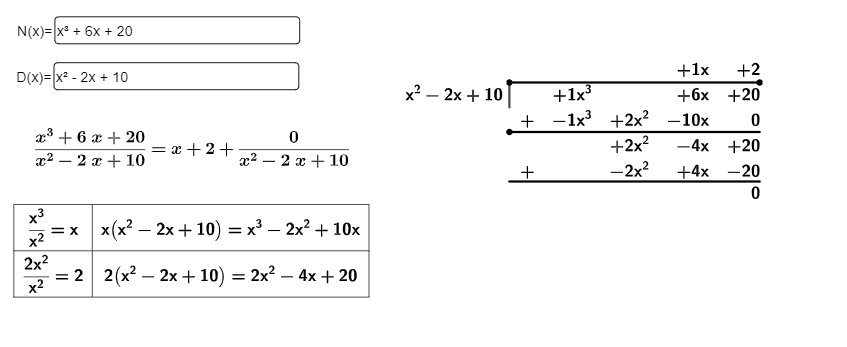# Adding leading zeros from the second row is the last in row to a matrix

jospercomp shared this question 2 years ago

Adding leading zeros from left the second row from the last in row to a matrix to equalize the number of columns in the rows when the column sizes are reduced by one less per row.

input: {{4,-6,0,0},{4,-6,0},{8,-12}}

output: {{4,-6,0,0},{0,4,-6,0},{0,0,8,-12}}

1 row = 0 zeros in left

2 row = 1 zeros in left

3 row = 2 zeros in left

.....

n row = n-1 ceros in leftyour input is'nt a matrix, try

Join({input(1)},Sequence( Join(Sequence(0,k,1,j-1),input(j)),j,2,3))2

your input is'nt a matrix, try

Join({input(1)},Sequence( Join(Sequence(0,k,1,j-1),input(j)),j,2,3))2

to make it work for arbitrary matrix sizes, just replace the "3" at the end by "length(input)"1

Join({input(1)},Sequence( Join(Sequence(0,k,1,j-1),input(j)),j,2,length(input))})

Join({{l3(1)}, Sequence(Join({Sequence(0, k, 1, j - 1), l3(j)}), j, 2, length(l3))})1

Each two rows add one zeros

1 and 2 rows -> 0 zeros

3 and 4 rows -> 1 zeros

5 and 6 rows -> 2 zeros ...

Join({{l15(1)}, Sequence(Join({Sequence(0, k, 1, floor(j - 1) / 2), l15(j)}), j, 2, Length(l15))})2

If you have a variety of different scenarios where the number of needed zeroes per line follows different rules, then - instead of making a new command for each version - you could just use a more general solution that fills each line with however many zeroes are needed.

Assuming that the first row has the maximal number of entries you can use

`output = Sequence(Join({Sequence(0, k, 1, Length(input(1)) - Length(input(j))), input(j)}), j, 1, Length(input))`

Or if it's not clear which input row is the longest, then you can use

```maxlen = Max(Zip(Length(L), L, input))
output = Sequence(Join({Sequence(0, k, 1, maxlen - Length(input(j))), input(j)}), j, 1, Length(input))```1

Thank you very much!!! Yes are coefficients of polinomial where the degree of polynomial decrease the first rows have the maximum degree.1

hm,

then you have a LGS to write in matrix form?

you may find some code https://www.geogebra.org/m/...1

I have "upper triangular matrix" in coefficient polynomial of polynomial long division method.2

Making a Matrix like

Substitute(({N(x)-N(0)+a N(0), D(x)-D(0)+a D(0)}), {x^3,x^2,x,a}=Identity(4) )

?1

What is "a" value?

The matrix is to centralize the terms with the tabletext command. The zeros that were missing on the left is to square the matrix to the same number of hills and the terms are aligned because the coefficients command only gives the highest term but the polynomials are reduced in the problem1

Hm,

I dont know what you exactly doing but reading your polynoms N,D with my last provided formula you get a full sized matrix with the coeffizents of your polynoms.

e.g {{1, 0, 6, 20}, {0, 1, -2, 10}}

why are zeros missing? nothing really needs to be adjusted reading a polynom with upper formula...

there are also the command Coefficents() too, but a little bit tricky to use...